copyright 1998-2018 by Mark Verboom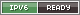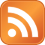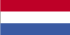Go back to Info

Power measurement procedure

This project started a long time ago when I borrowed Arno's G-Tech Pro meter.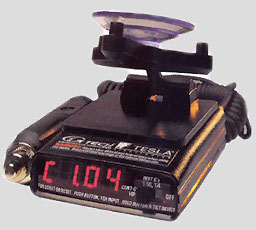I thought it was interesting to see how accurate the powermeasurements are this small device procedures.

The measurement

The basic idea is to measure how fast you can accelerate an object of a specific mass (the car). Based on this measurement you can calculate how much energy was required to accelerate the car which in turn can be converted to the amount of power.

The measurement consists of several parts:

• Measuring the weight
• Air and rolling resistance
• Relation engine RPM and speed
• Acceleration
Below is a short explanation per measurement.

Weight

To measure the weight I use a set of Longacre scales.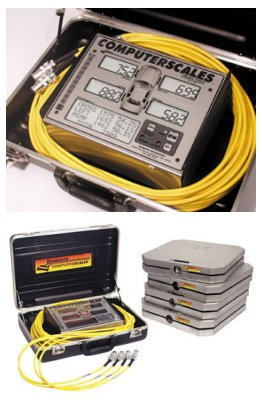Of course this is quite an expensive was to measure the weight (but very accurate). An alternative can be to use a caravan weighing scale.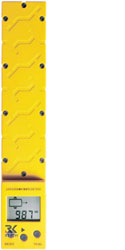Air and rolling resistance

To determine the air and rolling resistance, Race Technology created a coastdown procedure on their website. This procedure seems to work pretty well.

Using a stopwatch, several interval measurements can be done. The results can be put int the calculator on the Race Technology site and the values are calculated.

Relation engine RPM and speed

This is a very simple measurement when the car has a rev counter. Put the car in the same gear the acceleration measurement is done in. Drive the car at different speeds and record speed and engine RPM. Each measurement should result in the same ratio.

Acceleration

The Gtech-Pro this whole project started with can't be used to make graphs. Originally I bought an AP-22 from Race Technology to do this. This box can log the acceleration to the internal memroy.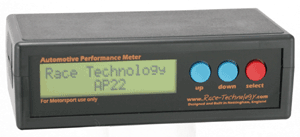Later I upgraded the AP-22 to a DL1, also from Race Technlogoy. This unit has much more options and greater accuracy. It uses a 5Hz built in GPS, acceleration sensors and can log the data to a CF card.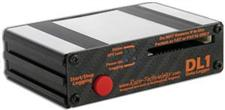During the acceleration measurement, the DL1 is fitted to the car. Then a number of measurements are done in 2nd or 3rd gear, from low RPM (just above idle) to the rev limiter.

Processing the data

The data logged by the DL1 is loaded into the (free) Analysis program from Race Technology. The other measurements (weight, air and rolling resistance) are also put into the software. It then calculates the power that was required to accelerate the car.

The data from the Analysis software is then exported to a CSV file and imported into a bit of software I wrote myself. This uses the engine RPM to car speed ratio to determine the engine RPM. Using the power and RPM it is possible to calculate the torque. Using these three variables GNUPlot draws the power graph.